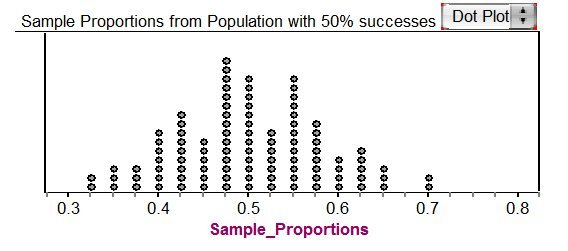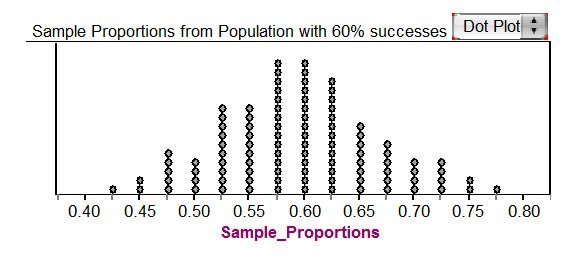# Election Poll, Variation 1

Alignments to Content Standards: 7.SP.A

Members of the seventh grade math group have nominated a member of their group to be class president. Every student in seventh grade will cast a vote. There are 2 candidates in the race and a candidate needs at least 50% of the votes to be elected. The math group wants to conduct a survey to assess their candidate’s prospects. There are almost 500 students in the seventh grade at their school. They do not have the resources to interview all seventh graders so they have decided to interview a sample of 40 seventh graders. They will obtain the seventh grade list of names from their school principal’s office and select the sample from this list. They plan to ask each student in the sample whether they plan to vote for their candidate or the other candidate.

1. How should the students select the sample of 40 to have the best chance of obtaining a representative sample? Describe clearly how they could use the random number table provided below to select the sample of 40 students. "Clearly" means that someone other than you could duplicate the sampling process by following your description.
2.3. Suppose that all 40 students selected from the list of seventh graders in the school respond to the survey, and the results showed that 18 students would vote for the math group’s candidate. In order to get elected, a candidate must receive at least 50% of the votes. Some members of the math group believe that on the basis of this sample outcome it is unreasonable to think that their candidate can win. Others in the group believe that it is possible that their candidate might win. Based on the initial survey results, should the math group students be discouraged, or is it reasonable to think their candidate might win? Justify your response statistically.

## IM Commentary

This task introduces the fundamental statistical ideas of using data summaries (statistics) from random samples to draw inferences (reasoned conclusions) about population characteristics (parameters). In the task built around an election poll scenario, the population is the entire seventh grade class, the unknown characteristic (parameter) of interest is the proportion of the class members voting for a specific candidate, and the sample summary (statistic) is the observed proportion of voters favoring the candidate in a random sample of class members. There are three variations of this task. Variations 2 and 3 have extensive commentary describing how they differ from this variation.

There are two important goals in this task: seeing the need for random sampling and using randomization to investigate the behavior of a sample statistic. These introduce the basic ideas of statistical inference, and can be accomplished with minimal knowledge of probability.

Random sampling (like mixing names in a hat and drawing out a sample) is not a new idea to most students, although the terminology is likely to be new. Most students readily grasp this as a fair way to select the sample because everyone gets an equal chance of being selected. Standard 1 uses the term “representative,” which has no technical definition in statistics and might be interpreted in terms of fairness. Students should understand that most samples, even if randomly selected, would not have exactly the same characteristics as the population from which they came.

Using simulation to repeatedly select random samples from a population with a specified proportion of successes will be a new idea to most students. Some discussion should revolve around this seemingly backward statistical notion of first specifying a population and then seeing if it could have produced the observed result as a reasonably likely outcome. Specifying the population structure allows the use of probability to determine the likelihood of the observed sample, and that is the basis of drawing statistical conclusions.

## Solutions

Solution: Solution to part (a)

The sample of 40 students should be selected randomly so that all groups of 40 students have an equal chance of being selected. This is a fair way to do the sampling and, moreover, one that allows the questions in part b to be answered. The randomization can be done by numbering the students and then selecting 40 distinct random numbers over that range to be the sampled students.

Solution: Solution to part (b)

Simulation provides a sound method for determining whether or not a sample proportion of 18/40 = 0.45 can reasonably be obtained from a population with 50% (0.50) “successes.” One way to accomplish this is to randomly sample 40 items, with replacement, from a group of items that have 50% marked as “success.” This can be done mechanically by sampling from 5 red and 5 white chips in a bag, or electronically by sampling from a list of five 1’s and five 0’s in a computer or graphing calculator. Each sample of 40 outcomes produces one simulated sample proportion of “successes”; the process is then repeated many times to generate a distribution of sample proportions.

Here is a simulation resulting in 100 sample proportions for samples of size 40 each. Notice that the observed result of 0.45 is not very far out in the lower tail; this value or a smaller value occurs 30 times out of the 100 trials. Thus, the students should not be discouraged; it is reasonable to think that 50% of the class might vote for their candidate.The plot below is the result for a similar simulation with 60% successes in the population. Here, the observed 0.45 is far out in the left tail, with only 3 of 100 trials being that small or smaller. Based on this survey result, it is not reasonable to think that 60% of the students would vote for their candidate.